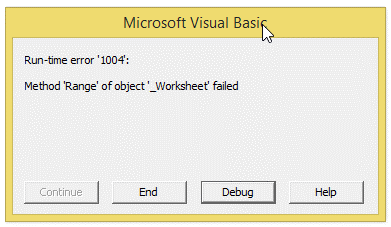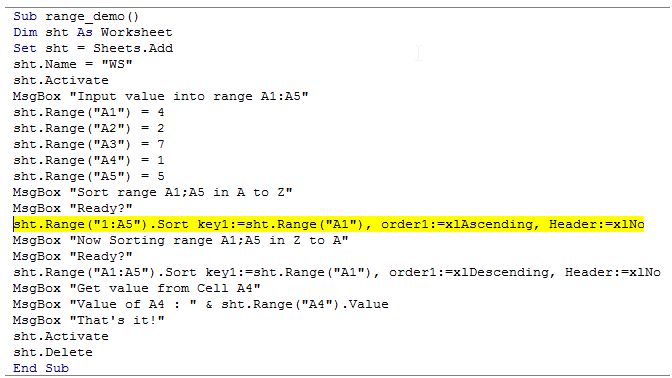# Excel Made Easy

## Vba macro debugging in Excel

Debugging is a most common and most often used word in the world of programming and Excel VBA is no exception.

Debugging is a process of idenfying errors in a program so as to rectify it for proper execution of the program.

"Debugging" came from the word "bug" which usually indicates flaws or defects and the term "Debug" signifies, removal of bugs.

There are a number Debugging techniques such as following:

1.Interactive debugging

2.Control flow

3.Integration testing

4.Log file

5.Unit testing

The VBA code is debugged from Top to Bottom and is sort of, Control flow analysis.

The debugging is used to spot out the error.

In large programs, it�s the Degugging, that saves us from identifying the flaws with less effort.

Consider the following code:

Please note that there is an error in the below mentioned code which when run returns an error, which when not handled properly will result in crashing of this workbook.So this code is not meant to run for an amateur programmer.

1. Sub range_demo()
2. Dim sht As Worksheet
3. Set sht = Sheets.Add
4. sht.Name = "WS"
5. sht.Activate
6. MsgBox "Input value into range A1:A5"
7. sht.Range("A1") = 4
8. sht.Range("A2") = 2
9. sht.Range("A3") = 7
10. sht.Range("A4") = 1
11. sht.Range("A5") = 5
12. MsgBox "Sort range A1;A5 in A to Z"
14. sht.Range("A1:A5").Sort key1:=sht.Range("A1"), order1:=xlAscending, Header:=xlNo
15. MsgBox "Now Sorting range A1;A5 in Z to A"
17. sht.Range("1:A5").Sort key1:=sht.Range("A1"), order1:=xlDescending, Header:=xlNo
18. MsgBox "Get value from Cell A4"
19. MsgBox "Value of A4 : " & sht.Range("A4").Value
20. MsgBox "That's it!"
21. sht.Activate
22. sht.Delete
23. End Sub

The above code when run will result in error:(Wrong Range in the highlighted text above) and displays a dialog box as shown below:When Debug is clicked, it will highlight the line containing error as shown below: